# JP4181310B2 - Formula recognition apparatus and formula recognition method - Google Patents

## Info

Publication number
JP4181310B2
JP4181310B2 JP2001063968A JP2001063968A JP4181310B2 JP 4181310 B2 JP4181310 B2 JP 4181310B2 JP 2001063968 A JP2001063968 A JP 2001063968A JP 2001063968 A JP2001063968 A JP 2001063968A JP 4181310 B2 JP4181310 B2 JP 4181310B2
Authority
JP
Japan
Prior art keywords
character
relationship
formula
characters
mathematical expression
Prior art date
Legal status (The legal status is an assumption and is not a legal conclusion. Google has not performed a legal analysis and makes no representation as to the accuracy of the status listed.)
Active
Application number
JP2001063968A
Other languages
Japanese (ja)
Other versions
JP2002269499A (en
Inventor

Original Assignee

Priority date (The priority date is an assumption and is not a legal conclusion. Google has not performed a legal analysis and makes no representation as to the accuracy of the date listed.)
Filing date
Publication date
Application filed by 東芝ソリューション株式会社, 昌和 鈴木 filed Critical 東芝ソリューション株式会社
Priority to JP2001063968A priority Critical patent/JP4181310B2/en
Publication of JP2002269499A publication Critical patent/JP2002269499A/en
Application granted granted Critical
Publication of JP4181310B2 publication Critical patent/JP4181310B2/en
Application status is Active legal-status Critical
Anticipated expiration legal-status Critical

## Images

•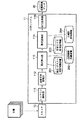•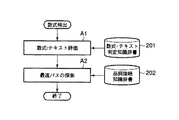•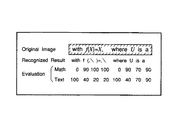•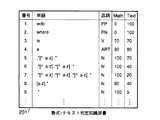•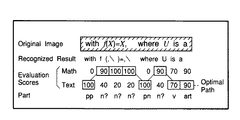•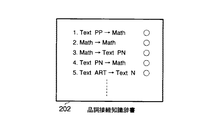•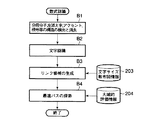•••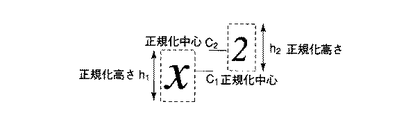•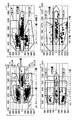•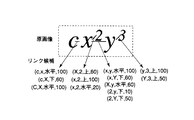•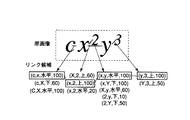•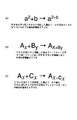## Classifications

• GPHYSICS
• G06COMPUTING; CALCULATING; COUNTING
• G06KRECOGNITION OF DATA; PRESENTATION OF DATA; RECORD CARRIERS; HANDLING RECORD CARRIERS
• G06K9/00Methods or arrangements for reading or recognising printed or written characters or for recognising patterns, e.g. fingerprints
• G06K9/62Methods or arrangements for recognition using electronic means
• G06K9/68Methods or arrangements for recognition using electronic means using sequential comparisons of the image signals with a plurality of references in which the sequence of the image signals or the references is relevant, e.g. addressable memory
• G06K9/6807Dividing the references in groups prior to recognition, the recognition taking place in steps; Selecting relevant dictionaries
• G06K9/6814Dividing the references in groups prior to recognition, the recognition taking place in steps; Selecting relevant dictionaries according to the graphical properties
• G06K9/6835Discrimination between machine-print, hand-print and cursive writing
• GPHYSICS
• G06COMPUTING; CALCULATING; COUNTING
• G06KRECOGNITION OF DATA; PRESENTATION OF DATA; RECORD CARRIERS; HANDLING RECORD CARRIERS
• G06K9/00Methods or arrangements for reading or recognising printed or written characters or for recognising patterns, e.g. fingerprints
• G06K9/62Methods or arrangements for recognition using electronic means
• G06K9/72Methods or arrangements for recognition using electronic means using context analysis based on the provisionally recognised identity of a number of successive patterns, e.g. a word
• GPHYSICS
• G06COMPUTING; CALCULATING; COUNTING
• G06KRECOGNITION OF DATA; PRESENTATION OF DATA; RECORD CARRIERS; HANDLING RECORD CARRIERS
• G06K2209/00Indexing scheme relating to methods or arrangements for reading or recognising printed or written characters or for recognising patterns, e.g. fingerprints
• G06K2209/01Character recognition

## Description


BACKGROUND OF THE INVENTION
The present invention relates to a mathematical expression recognition apparatus, a mathematical expression recognition method, a character recognition apparatus, and a character recognition method used for recognizing a document image including mathematical expressions.

[Prior art]
Although there are not many reports on character recognition of printed documents including mathematical expressions, they have been performed little by little. In character recognition in this field, characters are not arranged one-dimensionally, but are arranged two-dimensionally, such as subscripts, powers, and up and down fraction lines. Therefore, not only the character recognition result of each character but also means for determining position information in the mathematical formula such as where the character is in the subscript, power, denominator / numerator, etc. Therefore, when this character recognition is performed by a computer, the time required for the processing is longer than that of normal character recognition that does not target mathematical expressions.

Examples of reports that enable this to be processed in a practical time include the methods described in   and  shown below. These describe the relationship between the upper and lower characters of a character in a mathematical expression as a rule, and realize the mathematical expression recognition by determining the position of a normal character, subscript, power, denominator / numerator, and the like.

 Masayuki Okamoto, Towa Kyung Domusafirihashim, "Recognition of Mathematical Structure Using Peripheral Distribution Features", IEICE Transactions, J78-DII, No.2, pp.366-370 (1995)
Reference  Masayuki Okamoto, Hiroyuki Higashi, “Recognition of Mathematical Structure Focusing on Symbol Layout”, IEICE Transactions, J78-DII, No.3, pp.474-482 (1995)
Reference  RJ Fateman, T. Tokuyasu, BP Berman and N. Mitchell, "Optical Character Recognition and Parsing of Typeset Mathematics," Journal of Visual Communication and Image Representation, Vol 7, No. 1, pp.2-15 ( 1996)

[Problems to be solved by the invention]
However, in the prior art described above, the position of a character is determined based on local features such as a normal character, a subscript, a power, a denominator / numerator, and so on. There were problems such as greatly affecting the judgment. For example, if a normal character that exists in a certain place is mistakenly determined as a subscript, all of the subsequent normal characters that are lined up on the same horizontal position as the erroneously determined character will be appended. In some cases, a phenomenon such as being erroneously determined as a character existing in the character area may occur. That is, local misrecognition greatly disrupts the overall structural recognition of the mathematical formula.

Further, the above-described technique is mainly related to character recognition inside a mathematical expression, and the method of detecting a mathematical expression appearing in text relies on a mechanism such as simply searching for a symbol.

Accordingly, the present invention has been made to solve the above problem, and provides a mathematical expression recognition apparatus, a mathematical expression recognition method, a character recognition apparatus, and a character recognition method capable of recognizing a mathematical expression with high accuracy from a document including mathematical expressions. The purpose is to provide.

[Means for Solving the Problems]
In order to solve the above-described problems, the mathematical expression recognition apparatus according to the present invention can correspond to text and mathematical expressions for each word type that can be identified by a regular expression and character recognition means that performs character recognition of a document image including mathematical expressions. A first knowledge dictionary that defines evaluation values each indicating gender, and a reference to the first knowledge dictionary, and each word included in the character recognition result obtained by the character recognition means corresponds to a text and a mathematical expression, respectively. To connect words while selecting either text or mathematical expression for each word based on means for obtaining the evaluation value and the formal grammar and the evaluation value of each text and mathematical expression calculated for each word And a mathematical expression detecting means for detecting a word corresponding to the mathematical expression based on the search result.

In this mathematical expression recognition device, when the mathematical expression area is recognized by normal character recognition, various words included in the character recognition result by the regular expression are classified into the types in consideration of the appearance of various unexpected characters as the recognition result. A knowledge dictionary is prepared for acquiring evaluation values that are classified every time and that indicate the possibility of corresponding to a text and a mathematical expression for each classified word type. By using this knowledge dictionary, it is possible to give an evaluation value to each word flexibly. Formula detection is based on formal grammar, text calculated for each word, and the evaluation value of each formula. The best path to connect words while selecting either text or formula for each word. Is done by exploring. As a result, the mathematical formula area can be detected with high accuracy, so that the mathematical formula can be recognized with high accuracy from the document including the mathematical formula.

The mathematical expression recognition apparatus according to the present invention includes a character recognition unit that performs character recognition of a document image including a mathematical expression, a unit that detects a mathematical expression region from the character recognition result obtained by the character recognition unit, and the preceding and following characters. Means for storing a plurality of pieces of sample information indicating the relationship between the normalized size and the center position between characters before and after the horizontal position, subscript, and superscript, for each character type And a relationship between the normalized size and its center position for each of the preceding and following characters included in the mathematical expression region, and based on the calculation result and sample information corresponding to the relationship between the character types of the preceding and following characters. Means for obtaining link candidates indicating the possibility of corresponding to the horizontal position relationship, subscript subscript relationship, and superscript subscript relationship for each of the preceding and following characters.

In this mathematical expression recognition device, a plurality of different pieces of sample information are prepared for the preceding and following character types, and the horizontal position relationship, the subscript relationship, and the superscript relationship correspond to the character types between characters to be determined. By referring to the sample information, it is possible to determine the horizontal positional relationship, the subscript relationship, and the superscript relationship with higher accuracy. Therefore, it is possible to greatly reduce the determination error itself relating to the position of the character in the mathematical expression, and to greatly improve the recognition efficiency of the mathematical structure.

The mathematical expression recognition apparatus according to the present invention includes a character recognition unit that performs character recognition of a document image including a mathematical expression, a unit that detects a mathematical expression region from the character recognition result obtained by the character recognition unit, and the preceding and following characters. Means for storing sample information indicating the relationship between the normalized size and the center position between the characters before and after the horizontal position, subscript, and superscript, and in the formula area Calculate the relationship between the normalized size and the center position for each of the included characters, and based on the calculation result and the sample information, the horizontal position relationship and the subscript relationship for each of the preceding and following characters. A character structure determining means for obtaining a link candidate composed of an intercharacter structure candidate that may be applicable in the superscript relation and its evaluation value; and a character height of each character included in the mathematical expression area Based on the means for storing a global evaluation condition determined in advance based on the distribution of the above, the global evaluation condition, and the link candidate, a horizontal positional relationship and a subscript relationship between the preceding and following characters , Searching for an optimal path for connecting the characters in the formula area without contradiction while selecting any inter-character structure candidate in the superscript relation, and based on the search result, between the characters And means for recognizing a horizontal positional relationship, a subscript subscript relationship, and a superscript subscript relationship.

In this way, not only the local relationship between characters is determined, but also the characters in the formula area are connected consistently so that the total evaluation value is finally maximized in consideration of global evaluation conditions. Therefore, even if an error occurs in the position determination between specific characters, it is possible to prevent that it affects the structure of the entire mathematical expression.

DETAILED DESCRIPTION OF THE INVENTION
Hereinafter, embodiments of the present invention will be described with reference to the drawings.
FIG. 1 shows the configuration of a character recognition system according to an embodiment of the present invention. The character recognition (OCR) system 11 is for recognizing a print document including a mathematical expression represented by, for example, a science and technology document. The recognition process is performed for each of the text area and the mathematical expression area, and digitized document data including mathematical expression data and text data is output as recognition result data 20. The document to be read is not only a printed document but also a document image mixed with mathematical formulas already converted into image data.

The OCR system 11 is realized as software executed on a computer, and its function modules include a layout analysis unit 111, a normal character recognition unit 112, a mathematical expression detection unit 113, a mathematical expression recognition unit 114, and an output as illustrated in FIG. A conversion unit 115, a mathematical expression / text determination knowledge dictionary 201, a part-of-speech connection knowledge dictionary 202, a character size scatter diagram information storage unit 203, and a global evaluation information storage unit 204 are provided. These dictionaries and storage units are stored in a storage medium such as a semiconductor memory or a magnetic disk.

The recognition process is performed in the following order: 1) scan of document image, 2) layout analysis process, 3) normal character recognition process, 4) mathematical expression detection process, 5) mathematical expression recognition process, and 6) output conversion process. The present embodiment is particularly characterized in a method for realizing mathematical expression detection processing and mathematical expression recognition processing.

Before describing in detail the specific contents of the mathematical expression detection process and the mathematical expression recognition process, an outline of the flow of processing will be described first.

First, a printed image including a mathematical expression is read by the scanner device 10 to obtain a page image including the mathematical expression. Next, layout analysis is performed by the layout analysis unit 111, and the page image is divided into a figure, a table, and a text area. Then, the normal character recognition process by the normal character recognition unit 112 is performed on the sentence area. In this normal character recognition process, line segmentation and character segmentation based on a histogram are performed, and character recognition in units of one character is performed. Thereafter, the mathematical expression detection process by the mathematical expression detection unit 113 and the mathematical expression recognition process by the mathematical expression recognition unit 114 are performed based on the character recognition result.

In the mathematical expression detection process by the mathematical expression detection unit 113, the mathematical expression / text determination knowledge dictionary 201 and the part of speech connection knowledge dictionary 202 are used. The mathematical expression / text determination knowledge dictionary 201 defines, for each type of word that can be specified using a regular expression, an evaluation value that indicates the possibility of corresponding to a text and a mathematical expression. By referring to the mathematical expression / text determination knowledge dictionary 201, an evaluation value for each of the text and the mathematical expression is obtained for each word.

The part-of-speech connection knowledge dictionary 202 defines a formal grammar, in which rules for the connection relationship between a text part-of-speech and a mathematical expression are defined. Based on the formal grammar given by the part-of-speech connection knowledge dictionary 202 and the “evaluation value” for each of the text and the mathematical expression obtained by referring to the mathematical expression / text determination knowledge dictionary 201, the optimum between words included in the character recognition result By determining an appropriate connection relationship, the character recognition result is divided into a mathematical expression area and a text area.

All characters, symbols, and the like included in the formula area are sent to the formula recognition unit 114, where the formula structure recognition processing is performed. In this formula structure recognition process, a formula is decomposed into its constituent elements, and then the relationship between the horizontal position, the subscript, and the superscript is checked for each formula element. Here, a plurality of types of character size scatter diagrams (to be described later) stored in the character size scatter diagram information storage unit 203 and a global evaluation condition to be described later stored in the global evaluation information storage unit 204 are used. It is done. The character size scatter chart, which is sample information, shows the distribution of the normalized size and the center position between the preceding and following characters when the previous and next character pairs are in a horizontal position, subscript, and superscript relationship. It shows the situation. By referring to this character size scatter diagram, the inter-character structure candidate that may be applicable in the horizontal position relationship, subscript subscript relationship, or superscript subscript relationship for each character included in the formula element And a link candidate consisting of the evaluation value.

The global evaluation condition is a conditional expression for determining an appropriate inter-character structure based on a global evaluation regarding all characters included in the mathematical expression element. By using this global evaluation condition, not only the local relationship between each character is determined, but also the global evaluation condition is taken into account so that the characters in the formula element can be related to each other without any contradiction. A process for searching for the optimum route is performed.

The output conversion unit 115 performs a process of combining the recognition results and the like for each of the text area and the mathematical expression area and outputting the recognition result data 20.

(Formula detection method)
Hereinafter, a specific method of the mathematical expression detection process will be described.
In the present embodiment, as shown in FIG. 2, the mathematical expression area is detected by a mathematical expression detection method including the following two steps (A1, A2). This detection method is basically intended for detecting mathematical formulas from English documents.

<Step A1: Formula / Text Evaluation Processing>
In this step, each word is evaluated as a mathematical expression “Math” / text “Text” from the result obtained by normal character recognition. Here, the “word” refers to a character string delimited by a space as a recognition result. FIG. 3 illustrates this method.

The first line of FIG. 3 shows an example (Original Image) of an image actually input to the system 11. The second line is a result of normal character recognition by the normal character recognition unit 112 (Recognized Result). In the normal character recognition process of the present embodiment, a function for recognizing a mathematical expression is not implemented. Therefore, when a mathematical expression appears, the recognition result appears as various unexpected symbol strings. In this step A1, the recognition result is input, and each word is evaluated as a mathematical expression “Math” and a text “Text”. The values indicated as “Math” and “Text” in the two lines below the recognition result indicate examples of the result of evaluating each word in this way. In the present embodiment, this processing is performed by searching the mathematical expression / text determination knowledge dictionary 201 described above. FIG. 4 shows an example of data in the mathematical expression / text determination knowledge dictionary 201.

In FIG. 4, the line indicated by the number 1 indicates that the part of speech of the word spelled “with” is the preposition (PP), the evaluation value as “Math” (formula) is 0, and “Text” (text). The evaluation value is 100. Similarly, in the line indicated by the number 2, the part of speech of the word spelled “where” is a pronoun (PN), the evaluation value as “Math” is 0, and the evaluation value as “Text” is 100. It shows that. The line indicated by the number 3 indicates that the part of speech of the word spelled “is” is a verb (V), the evaluation value as “Math” is 70, and the evaluation value as “Text” is 70. . The line indicated by the number 4 indicates that the part of speech of the word spelled “a” is the article (ART), the evaluation value as “Math” is 90, and the evaluation value as “Text” is 90. . In this way, in the mathematical expression / text determination knowledge dictionary 201, for almost all words normally used in science and technology documents and the like, evaluation values relating to the spelling (arrangement of character codes), parts of speech, mathematical expressions, and text are stored in advance. It is registered.

Furthermore, in this embodiment, considering that the recognition result for the mathematical expression appears as various unexpected symbol strings, the regular expression can flexibly cope with various symbol strings. Regular expressions are words that can be expressed more flexibly and are usually used in search systems and the like. In this case, each symbol in the regular expression represents the following meaning.

That is, the line indicated by the number 5 in FIG. 4 indicates that the word includes characters other than a to z, that is, one character including a certain symbol. The part of speech of this word is a noun (N), which indicates that the evaluation value as “Math” is 100 and the evaluation value as “Text” is 70. Similarly, the line indicated by the number 6 indicates that the word includes two characters other than a to z, the part of speech is a noun (N), the evaluation value as “Math” is 100, “Text” The evaluation value is “40”. The line indicated by the number 7 indicates that the word contains three characters other than symbols a to z, the part of speech is a noun (N), the evaluation value as “Math” is 100, and “Text” The evaluation value is 20. The line indicated by the number 8 indicates one alphabetic character from a to z, the part of speech is a noun (N), the evaluation value as “Math” is 90, and the evaluation value as “Text” is 40. . The part of speech of the noun (N) indicates that the corresponding word is text.

By searching the mathematical expression / text determination knowledge dictionary 201 shown in FIG. 4 in the order of line numbers, the part-of-speech type and evaluation values for “Math” and “Text” are obtained for each word obtained as a result of character recognition.

That is, as shown in FIG. 3, for the word [with], the evaluation value as “Math” is 0 and the evaluation value as “Text” is 100 based on the knowledge of number 1 in FIG. For the word [f 1], the evaluation value as “Math” is 90 and the evaluation value as “Text” is 40 based on the knowledge of number 8 in FIG. For the three characters of the word [(, \], according to the rule of number 7, the evaluation value as “Math” is 100 and the evaluation value as “Text” is 20. Subsequently, the word [) =, \ ] Indicates that the evaluation value as “Math” is 100 and the evaluation value as “Text” is 20. However, this example is not shown in FIG. Similarly, for the word [where], the evaluation value as “Math” is 0 and the evaluation value as “Text” is 100 based on the knowledge of number 2 in FIG. The word [U] is evaluated to have an evaluation value of 90 as “Math” and 40 as an evaluation value of “Text” based on the knowledge of number 8 in FIG. Similarly, for the word [is], the evaluation value as “Math” is 70 and the evaluation value as “Text” is 70 based on the knowledge of number 3 in FIG. Also, since the knowledge of number 4 in FIG. 4 is preferentially applied to the last word [a] over the knowledge of number 8, the evaluation value as “Math” and the evaluation value as “Text” are both obtained as 90. It is done.

<Step A2: Search for Optimal Path>
In the next step A2, a process of searching for an optimal path from the evaluated result and connecting it is performed. FIG. 5 shows this state. In this step A2, the part-of-speech connection knowledge dictionary 202 which indicates which part of speech of the text can be connected to which part of speech and which part of speech of the text can be connected to the mathematical formula is used. FIG. 6 shows an implementation example of the part-of-speech connection knowledge dictionary 202.

In FIG. 6, “Text PP → Math” on the first line indicates that the text preposition (PP) can be connected to the following mathematical expression. Also, “Math → Math” on the second line indicates that mathematical expressions can be connected. “Math → Text PN” on the third line indicates that the mathematical expression can be connected to the pronoun (PN) of the subsequent text. “Text PN → Math” on the fourth line indicates that the text pronoun (PN) can be connected to the following mathematical expression. “Text ART → Text N” on the fifth line indicates that the article of the text (ART) can be connected to the noun (N) of the subsequent text.

All combinations that can be connected are registered in the part-of-speech connection knowledge dictionary 202, and other combinations cannot be connected.

In the search for the optimum path, only possible connections are traced for each word while selecting an expression “Math” / text “Text” according to the formal grammar rules of the part-of-speech connection knowledge dictionary 202 while adding evaluation values. It is done. In this way, among all the connectable routes, the route having the highest sum of the mathematical expression / text evaluation values is searched. Simply put, for example, in FIG. 5, as a connectable path from the word [with] to the next word [f], the word [with] “Math” and the word [f] “Math” and the word [ f] "Text" exists, and from the word [with] "Math", the word [f] "Math" and the word [f] "Text" exist, but the total evaluation of the selected path The route from the “Text” of the word [with] to the “Math” of the word [f] is selected so that the value becomes the highest. In FIG. 5, “Text”, “Math”, “Math”, “Math”, “Text” are the optimum paths for connecting the eight words from the first word [with] to the last word [a]. , "Math", "Text", and "Text" routes have been searched.

This search algorithm can be realized by beam search (or width-first search). Beam search is a well-known algorithm used in dynamic programming, etc., and in dynamic programming, the search space is determined by excluding those that are determined to be less likely to be optimal paths from the subsequent processing. Is an efficient method that can simultaneously reduce the amount of computation and the amount of memory.

As a result of the above search processing, it is determined whether each word is a mathematical expression “Math” / text “Text”, and a mathematical expression area and a text area can be detected. In FIG.
f
(, \
) =, \
U
It can be seen that all the other words are determined as the text “Text”. The area in the image data corresponding to the word determined as the mathematical expression “Math” is the mathematical expression area, and the area in the image data corresponding to the word determined as the text “Text” is the text area.

In this example, the connection is checked using parts of speech, so it is equivalent to describing the grammar in regular grammar, but in reality the connection relationship is described in a more advanced formal grammar such as context-free grammar. You can also

In conventional systems, if a recognition result includes a symbol that appears to be a mathematical expression such as parentheses or italics, it is often determined by a simple rule such as determining it as a mathematical expression. Therefore, various symbols that appear as recognition results when a mathematical expression is recognized cannot be dealt with. For example, if the word [a] exists in a document, it is determined whether it is an article or a mathematical expression. Was virtually impossible. In the present embodiment, as described above, by checking the evaluation value of each word, it is possible to more accurately determine whether each word is the mathematical expression “Math” or the text “Text”. In addition, since the formal grammar is checked, for example, the text [a], which is the article, can only be followed by a text noun. Is also possible.

(Formula recognition method)
Compared with normal character recognition, mathematical expression recognition requires a method for examining structures such as subscripts, powers, and denominator numerators in addition to recognition of characters themselves. Among these, in this embodiment, the same method as the conventional character recognition is used for the recognition of the character itself. The method for examining the mathematical structure is performed by the following four steps (B1, B2, B3, B4) as shown in FIG.

<Step B1: Structure detection of denominator numerator, left subscript, accent, root number, dots, etc.>
In this step, fraction lines and root signs are detected from the image data in the mathematical formula area, and the denominator numerator, the inside of the root sign, etc. are decomposed into disjoint expressions. Similarly, left subscripts, accent symbols, points, etc. are detected and deleted from the image data in the formula area.

For example, when the mathematical expression as shown in FIG. 8 is included in the mathematical expression area detected as described above, it is decomposed into four mathematical formula components as indicated by dotted lines, and the left suffix for each mathematical formula component. Delete ( 3 a → a), deletion of accent marks such as ^, ~, etc. on characters (xdx ^ → xdx), and deletion of root sign (√a + b → a + b) not shown in FIG. Delete point (x → x) etc. are performed.

Judgment of mathematical elements such as denominator numerator, left subscript, accent symbol, root number, point, etc. is done relatively accurately in the literature such as    above, and in many cases It can be determined by a determination formula based on the local positional relationship. Therefore, by performing these detection operations in advance by a simple determination method, the processing in subsequent steps B2 to B4 can be limited to, for example, processing related to subscripts and superscripts (powers). There is an advantage that processing can be speeded up.

<Step B2: Character recognition>
Subsequent steps B2 to B4 are performed on the partial mathematical expressions processed in step B1 that do not include any more fraction lines, accent symbols, left subscripts, root signs, points, or the like.

First, in step B2, black connected components are extracted from the partial mathematical image data obtained in step B1, and character recognition is performed for each black connected component. As a result, candidate characters as shown in FIG. 9 are obtained. FIG. 9 shows cx 2 y 3 This is an example in the case of character recognition of the image data of the partial mathematical expression. By this character recognition, uppercase letters, lowercase letters, etc. are obtained as candidate characters for each character (black connected component).

<Step B3: Generation of Link Candidate>
In the next step B3, the connection possibility of each character is examined for all of the obtained candidate characters using the relationship shown in FIG.

FIG. 10 shows values (normalized size and center position) used to determine whether the relationship between the front and rear two characters corresponds to a horizontal positional relationship, a subscript relationship, or a superscript relationship. It is a thing. In the figure, values indicated by h1 and h2 are normalized heights (normalized sizes) of the corresponding characters, respectively. The normalized size is obtained by correcting the size of characters on the same line so that they have the same size (height).

Here, the height of the whole character including the ascender part (for example, the character [d]) and the descender part (for example, the character [y]) is set as the normalized size. That is, h1 indicates the character height when “d” and “y” are overlaid at the position of the character. “D” is a character whose black connecting line segment extends to the upper limit of the ascender portion, and “y” is a character whose black connecting line segment extends to the lower limit of the descender portion. For example, “x” shown in the figure is shorter than “d” and “y”. Therefore, the normalized size h1 when “d” and “y” are overwritten can be obtained by multiplying the actual character height of “x” by a certain factor. The magnification value for obtaining the normalized size is individually defined for each character type, and the normalized size is obtained by multiplying the actual character size by the magnification. For example, for a lowercase letter “c”, a scaling factor is used so that the character height spreads in the vertical direction, and for a capital letter “C”, a scaling factor that spreads the character height only in the downward direction is used. become.

Similarly, for the character “2” in the subscript area, the normalized size h2 is obtained by multiplying the actual character size by the magnification corresponding to the character “2”. Usually, the actual size of the character that exists in the subscript area is smaller than the character that exists on the baseline, so it exists in the subscript area rather than the normalized size h1 of the character “x” that exists on the baseline. The normalized size h2 of the character “2” to be smaller becomes smaller.

In FIG. 10, c1 and c2 are normalization centers, respectively. The normalization center is the center position corrected so that the characters on the same line have the same height center position. Here, the center y coordinate of the circumscribed rectangle surrounding the normalized character size is used as the normalization center. And Now, let h1, c1, h2, and c2 be the normalized height and center coordinates of adjacent characters, respectively.
Normalized size relationship H = (h2 / h1) × 1000
Normalization center relationship D = {(c1-c2) / h1} × 1000
11 is plotted, the scatter diagram of FIG. 11 is obtained.

The four scatter diagrams (sample information) in FIGS. 11A to 11D are in a relationship of a pair of characters in a horizontal position, a pair of characters in a superscript relationship, and a subscript. The result of measuring the relationship between the normalized size and the normalized center (HD) for each pair of characters for each character type is shown. FIG. 11A is a scatter diagram when two consecutive characters are alphabets. Here, alphabets indicate alphabets, Greek letters, and numbers. Similarly, FIG. 11B shows the case where the alphabet and the operator move back and forth, FIG. 11C shows the case where the integral and the alphabet move back and forth, and FIG. The case where alphabets are mixed is shown.

Therefore, H and D are calculated for each candidate character examined in step B2, and it is evaluated to which of the polygonal regions shown on the scatter diagram corresponding to these character types H and D belong. Thus, it is possible to obtain a pair of inter-character structure candidates and their evaluation values (herein referred to as link candidates) that may be applicable in the horizontal position relationship, subscript relationship, and superscript relationship. For example, when the normalized size / normalized center relationship (HD) between two characters before and after is included in the polygonal regions P1 and P2 in FIG. 11A, they are superscripted. (Evaluation value is higher when P1 is included than P2). When included in the polygonal regions P3 and P4, they are evaluated as having a subscript relationship (the evaluation value is higher in the case of being included in P3 than in P4). When included in the polygonal regions P6 and P5, they are evaluated as having a horizontal positional relationship (the evaluation value is higher when included in P6 than in P5).

FIG. 12 shows the generated link candidates in an easy-to-understand manner. In FIG. 12, each link candidate represents (parent (left) candidate character, child (right) candidate character, connection type, evaluation value). Link candidates are generated for every two characters before and after, but link candidates are also generated for two characters before and after the character with a subscript area in between (the relationship between x and y in FIG. 12). The

As shown in FIG. 12, the link candidate between the character “c” and the character “x” can be obtained by referring to the scatter diagram of FIG.
(C, x, horizontal, 100)
(C, X, bottom, 60)
(C, X, horizontal, 100).

In this case, the set of (C, x) cannot be from the scatter diagram.

Further, the link candidate between the character “x” and the subscript character “2” can be obtained by referring to the scatter diagram of FIG.
(X, 2, upper, 60)
(X, 2, top, 100)
(X, 2, horizontal, 20).

Further, a link candidate between the character “x” and the character “y” in consideration of the subscript character “2” can be obtained by referring to the scatter diagram of FIG.
(X, y, horizontal, 100)
(X, Y, bottom, 60)
(X, y, horizontal, 60)
(2, y, bottom, 10)
(2, Y, bottom, 50).

Further, the link candidate between the character “y” and the subscript character “3” can be obtained by referring to the scatter diagram of FIG.
(Y, 3, top, 100)
(Y, 3, top, 50).

The present embodiment is characterized in that there are four scatter diagrams (sample information) shown in FIG. As shown in FIG. 11, the distribution of the relationship between characters varies considerably depending on the character type before and after. Therefore, in this embodiment, this figure is prepared for each character type of the preceding and following characters, and subscript determination is performed using a scatter diagram corresponding to the character types of the two characters to be determined.

In the above-mentioned documents   , the subscript determination is performed only by whether the normalized center position is around the center of the parent character or shifted vertically. In FIG. 11, it is understood that the subscript determination is performed using only the ordinate, and there is a considerable case of erroneous determination. On the other hand, in the present invention, determination is made by a scatter diagram in a two-dimensional region in combination with a ratio of sizes, and further it is determined by obtaining a scatter diagram in combination for each symbol type. The accuracy of subscript judgment is greatly improved.

Before explaining the next step, we will explain why formula structure recognition becomes an optimal path problem.

That is, the structure of the mathematical expression is represented by a tree structure, and the symbols are not arranged in a single column, so it is not understood why it becomes a problem of finding the optimum “path”. In the present invention, this is achieved by obtaining a spanning tree representing an optimal mathematical structure from the link network created in step B3. “Determining the spanning tree” means “determining the connection of each character to the parent character”. Therefore,
A set of (parent (left) candidate character, child (right) candidate character, connection type, evaluation value) is called a “link candidate”, and each character rectangle has all link candidates that have that character as children. Yes. In addition, if one link candidate is selected from each character rectangle, one spanning tree is determined. Since such a choice can be viewed as a “route”, it makes the theory that it becomes an optimal route problem.

<Step B4: Search for Optimal Path>
Next, in step B4, the link candidate generated for each character space in step B3 is traced from the back (or from the front) to search for the optimum route for connecting these link candidates. In other words, in consideration of the connection relationship between each character (horizontal position relationship, subscript relationship, superscript relationship), characters are inconsistent while selecting one of the link candidates between the previous and next characters. Among routes that can be connected without any difference, the route having the highest total evaluation value is examined. In this case, not only the local evaluation value for each character given by each link candidate, but also the global based on the character height distribution between the characters included in the corresponding mathematical component as shown below On the basis of the four global evaluation conditions, the route having the highest global evaluation value is determined as the optimum route.

1. The sum of the evaluation values of each link candidate in the route is used as a global evaluation value.

2. If the normalized size of characters in the subscript area is larger than the normalized size of each character, the global evaluation value is lowered. This corresponds to the case of FIG. That is, when the normalized size of the character determined to be present in the subscript area by the link candidate is equal to or larger than the normalized size of each of the other characters, the global evaluation value is lowered. In FIG. 14A, when “b” is determined to be the same size as the subscript, the character size of “b” is the same as “a”, so the global evaluation value is lowered.

3. If there is a character in the subscript area that is close to the same line as the character on the baseline, lower the global rating. That is, if a character on the baseline and a character that falls within the narrow region (P2, P4, P6) in the scatter diagram of FIG. 11 are in the subscript region, the global evaluation value is lowered. In FIG. 14B, when “x” is determined to be an uppercase “X”, the character “B” close to the same line as the baseline character “A” is in the subscript region, and the global evaluation value is lowered. .

4. If the normalized character size of the alphabets on the baseline varies more than a certain level, lower the global rating. This corresponds to the case of FIG. That is, when the alphabets on the baseline have different normalized sizes, the global evaluation value is lowered. FIG. 14C shows a case where “C” is erroneously determined to be lowercase “c”. In this case, the normalized size of “c” is larger than the normalized size of “A”, and the global evaluation value is Lower.

In this way, the global evaluation condition is that the characters in the formula are consistently selected while selecting link candidates of the horizontal position relationship, subscript relationship, or superscript relationship for each character before and after. This is a condition for correcting the total evaluation value in the connectable route with a global standard. As a search algorithm for searching for an optimum route with the highest global evaluation value, a beam search (or width-first search) can be used.

FIG. 13 shows an example of the optimum route determined in consideration of the global evaluation value. In this way, the optimum link candidate is selected for each character, and it is determined for each character whether the horizontal position relationship, subscript relationship, or superscript relationship is applicable.

In the method of the above-mentioned document   , there was no idea of such a global evaluation value as described above. Therefore, if a character on the baseline is mistaken for a subscript, There was a problem that all subsequent characters were subscripted. This is because the subscript / power determination of each character is calculated based only on local features. In contrast to this, in the present invention, since a global evaluation value is calculated when following a path, even if one character is mistakenly determined as a subscript, a phenomenon in which all subsequent characters are subscripted. It does not occur. In addition, this global evaluation value calculation method can be used to evaluate the result of mathematical expression recognition by an external device. This can also be applied to composite determination.

Then, by adding the left subscript, the accent symbol, the root sign, etc. deleted in step B1 to the character string for which the optimum connection between candidate characters is determined in this way, the final expression regarding the corresponding mathematical formula component is obtained. Recognition results can be obtained. By performing the processing of steps B2 to B4 for each formula component, the final recognition result regarding the formula area is obtained. And the recognition result data of the text area | region containing a numerical formula are obtained by synthesize | combining the recognition result regarding a numerical area with the recognition result of a text area.

As described above, according to the present embodiment, 1) while selecting either text or mathematical expression for each word based on the formal grammar and the evaluation value of each text and mathematical expression calculated for each word. By searching for an optimum route for connecting words, it is possible to detect a mathematical expression region with high accuracy. 2) By preparing multiple scatter plots showing the relationship between the normalized size and the center position between the preceding and following characters for each character type before and after, horizontal positional relationship, subscript relationship, superscript It becomes possible to determine the subscript relation. 3) Even if an error occurs in the position determination between specific characters by searching for the optimum path in consideration of the global evaluation condition as well as the determination of the local relationship between each character. It is possible to prevent it from affecting the structure of the entire mathematical formula. 4) A link is generated by performing a process of decomposing each mathematical expression component and detecting a left subscript, an accent symbol, a root sign, etc. from each mathematical expression component in advance as preprocessing for link candidate generation and optimum path search. Characters that are candidates for candidate generation can be reduced, and processing efficiency can be improved. The effect is obtained.

Since all the functions of the OCR system 11 according to the present embodiment can be realized by software, a program for causing a computer to execute the above-described processing procedures is prepared and stored in a computer-readable storage medium. The same effect as that of the present embodiment can be easily obtained simply by introducing the program into a computer and executing the program.

Further, the present invention is not limited to the above-described embodiment, and various modifications can be made without departing from the scope of the invention in the implementation stage. Further, the above embodiments include inventions at various stages, and various inventions can be extracted by appropriately combining a plurality of disclosed constituent elements. For example, even if some constituent requirements are deleted from all the constituent requirements shown in the embodiment, the problem described in the column of the problem to be solved by the invention can be solved, and the effect described in the column of the effect of the invention Can be obtained as an invention.

【The invention's effect】
As described above in detail, according to the present invention, it is possible to recognize a mathematical expression with high accuracy from a document including the mathematical expression, and it can be effectively used for, for example, digitization of scientific and technical documents.
[Brief description of the drawings]
FIG. 1 is a block diagram showing a functional configuration of an OCR system according to an embodiment of the present invention.
FIG. 2 is a flowchart showing a procedure of a mathematical expression detection method according to the embodiment.
FIG. 3 is an exemplary view for explaining a mathematical expression / text evaluation process performed by mathematical expression detection according to the embodiment;
FIG. 4 is a view for explaining an example of a mathematical expression / text determination knowledge dictionary used in the embodiment;
FIG. 5 is an exemplary view for explaining an optimal path search process performed in the mathematical expression detection according to the embodiment;
FIG. 6 is a diagram for explaining a part-of-speech connection knowledge dictionary used in the embodiment;
FIG. 7 is a flowchart showing a procedure of a mathematical expression recognition method in the embodiment.
FIG. 8 is a view showing a state of mathematical expression decomposition performed by mathematical expression recognition according to the embodiment;
FIG. 9 is a view for explaining a candidate character detection operation performed in mathematical expression recognition according to the embodiment;
FIG. 10 is a diagram for explaining a calculation process of a normalization size and a normalization center performed in mathematical expression recognition according to the embodiment;
FIG. 11 is a diagram for explaining a scatter diagram used in the embodiment;
FIG. 12 is a view for explaining link candidates generated for each continuous character in the embodiment;
FIG. 13 is an exemplary view for explaining optimum path search processing in mathematical expression recognition according to the embodiment;
FIG. 14 is a view for explaining conditions for calculating a global evaluation value used in formula recognition according to the embodiment;
[Explanation of symbols]
11 ... OCR system
111 ... Layout analysis unit
112 ... Normal character recognition unit
113... Formula detection unit
114. Formula recognition unit
115: Output converter
201 ... Math / text judgment knowledge dictionary
202 ... Part of speech connection knowledge dictionary
203 ... Character size scatter plot
204 ... Global evaluation information

## Claims (6)

1. A character recognition means for character recognition of a document image including a mathematical expression;
A first knowledge dictionary that defines an evaluation value for each word type that can be specified by a regular expression and that indicates a possibility of corresponding to a text and a mathematical expression;
Means for referring to the first knowledge dictionary to obtain an evaluation value corresponding to each of the text and the mathematical expression for each word included in the character recognition result obtained by the character recognition means;
Based on the formal grammar and the evaluation value of each text and mathematical formula calculated for each word, search for an optimal path for connecting words while selecting either text or mathematical formula for each word, Formula detection means for detecting a word corresponding to the formula based on the search result;
When the preceding and following characters are in the relationship of the horizontal position, the subscript, and the superscript, respectively, sample values indicating the relationship between the normalized size between the preceding and succeeding characters and the center position thereof, the normalized size and the Means for storing a plurality of pieces of sample information described on the scatter diagram with the center position as an axis for each character type;
For each of the preceding and following characters included in the equation detected by the equation detecting means, the relationship between the normalized size and its center position is calculated, and the calculation result corresponds to the relationship between the character types of the preceding and following characters. In the scatter diagram of information, the area where sample values between characters in a horizontal position relationship are distributed, the area where sample values between characters in a subscript relationship are distributed, and the sample value between characters in a subscript relationship It is possible to fall within the horizontal positional relationship, subscript subscript relationship, and superscript subscript relationship for each of the preceding and following characters included in the formula Intercharacter structure determination means for obtaining a link candidate consisting of a character intercharacter structure candidate and its evaluation value;
Globally for correcting the total evaluation value of the path of the intercharacter structure candidate that is determined in advance based on the distribution of the character height of each character included in the formula and can connect the characters in the formula without any contradiction When the normalized size of the characters included in the subscript area is equal to or larger than the normalized size of the characters included in the horizontal area, the total evaluation value is lowered, and between the characters included in the horizontal area Based on the global evaluation condition including at least one of the conditions of lowering the total evaluation value when the normalized size has a variation of a certain value or more, and the link candidate, the included in the formula While selecting the inter-character structure candidate of horizontal position relation, subscript relation, or superscript relation for each of the characters before and after, in a path that can connect the characters in the formula without contradiction Together By the evaluation value for searching for a route that maximizes comprises a formula recognition means for determining corresponds to any of the front and rear of the horizontal positional relationship for each inter-character, subscript relationship, superscript relationship The mathematical expression recognition apparatus characterized by the above-mentioned.
2. A second knowledge dictionary defining the relation between the text part of speech and the mathematical formula of each connectable word as the formal grammar;
The mathematical expression detecting means includes
In accordance with the part of speech for each word included in the character recognition result and the formal grammar given by the second knowledge dictionary, all the paths that can be connected between the words are selected while selecting either text or mathematical expression for each word. 2. The mathematical expression recognition apparatus according to claim 1, wherein the mathematical expression recognition apparatus selects and searches for an optimum path having a maximum total evaluation value regarding the text or mathematical expression of each word.
3. Further comprising means for decomposing the mathematical formula detected by the mathematical formula detection means for each mathematical formula component, detecting at least a left subscript, an accent symbol, a root number, and points from each mathematical formula component and excluding them. And
The inter-character structure determination unit, to the excluded equation components, formulas recognition apparatus according to claim 1, wherein the obtaining link candidates.
4. A character recognition step for character recognition of a document image including a mathematical expression;
For each word type that can be specified by a regular expression, refer to the first knowledge dictionary that defines an evaluation value indicating the possibility that it corresponds to a text and a mathematical expression, and the character recognition result obtained by the character recognition step is For each word included, a rating corresponding to the text and formula Gaining value,
Based on the formal grammar and the evaluation value of each text and mathematical formula calculated for each word, search for an optimal path for connecting words while selecting either text or mathematical formula for each word, A formula detection step for detecting words corresponding to the formula based on the search result;
When the preceding and following characters are in the relationship of the horizontal position, the subscript, and the superscript, respectively, sample values indicating the relationship between the normalized size between the preceding and succeeding characters and the center position thereof, the normalized size and the A plurality of sample information described on the scatter diagram with the center position as an axis for each character type before and after is prepared in advance, and normalized for each character before and after included in the formula detected in the formula detection step. The area where the sample values between the characters in the horizontal position relationship are distributed in the scatter diagram of the sample information corresponding to the relationship between the character types of the preceding and following characters. By evaluating whether a sample value between characters in a subscript relationship is distributed or an area in which sample values between characters in a subscript relationship are distributed A link consisting of candidate character structures that may be applicable in the horizontal positional relationship, subscript subscript relationship, superscript subscript relationship, and their evaluation values for each of the preceding and following characters included in the formula An inter-character structure determination step for obtaining candidates;
Globally for correcting the total evaluation value of the path of the inter-character structure candidate that is determined in advance based on the distribution of the character height of each character included in the formula and can connect the characters in the formula without any contradiction When the normalized size of the characters included in the subscript area is equal to or larger than the normalized size of the characters included in the horizontal area, the total evaluation value is lowered, and between the characters included in the horizontal area Based on a global evaluation condition including at least one of the conditions of lowering the total evaluation value when the normalized size has a variation of a certain value or more, and the link candidate, the included in the formula While selecting the inter-character structure candidate among the horizontal position relationship, subscript relationship, and superscript relationship for each character before and after, in the path that can connect the characters in the formula without any contradiction Together A mathematical expression recognition step for determining a horizontal positional relationship, a subscript relationship, or a superscript relationship for each of the preceding and following characters by searching for a route having the maximum evaluation value. The mathematical expression recognition method characterized by the above-mentioned.
5. The mathematical expression detecting step is performed for each word in accordance with a part of speech for each word included in the character recognition result, and a second knowledge dictionary that defines a relation between a text part of speech of each connectable word and a mathematical expression as the formal grammar. Select all the paths that can connect between words while selecting either text or mathematical expression, and search for the optimal path that maximizes the total evaluation value for the text or mathematical expression of each word. The mathematical expression recognition method according to claim 4.
6. Further comprising the step of decomposing the mathematical formula detected in the mathematical formula detection step for each mathematical formula component, detecting at least a left subscript, an accent symbol, a root number, and points from each mathematical formula component and excluding them. And
The mathematical expression recognition method according to claim 4, wherein the inter-character structure determining step obtains a link candidate for the excluded mathematical expression component.
JP2001063968A 2001-03-07 2001-03-07 Formula recognition apparatus and formula recognition method Active JP4181310B2 (en)

## Priority Applications (1)

Application Number Priority Date Filing Date Title
JP2001063968A JP4181310B2 (en) 2001-03-07 2001-03-07 Formula recognition apparatus and formula recognition method

## Applications Claiming Priority (4)

Application Number Priority Date Filing Date Title
JP2001063968A JP4181310B2 (en) 2001-03-07 2001-03-07 Formula recognition apparatus and formula recognition method
DE2002624128 DE60224128T2 (en) 2001-03-07 2002-03-05 Apparatus and method for recognizing characters and mathematical expressions
EP20020004377 EP1239406B1 (en) 2001-03-07 2002-03-05 Device and method for character recognition and for recognition of mathematical expressions
US10/087,772 US7181068B2 (en) 2001-03-07 2002-03-05 Mathematical expression recognizing device, mathematical expression recognizing method, character recognizing device and character recognizing method

## Publications (2)

Publication Number Publication Date
JP2002269499A JP2002269499A (en) 2002-09-20
JP4181310B2 true JP4181310B2 (en) 2008-11-12

# Family

## Family Applications (1)

Application Number Title Priority Date Filing Date
JP2001063968A Active JP4181310B2 (en) 2001-03-07 2001-03-07 Formula recognition apparatus and formula recognition method

## Country Status (4)

US (1) US7181068B2 (en)
EP (1) EP1239406B1 (en)
JP (1) JP4181310B2 (en)
DE (1) DE60224128T2 (en)

## Families Citing this family (60)

* Cited by examiner, † Cited by third party
Publication number Priority date Publication date Assignee Title
JP2007535740A (en) * 2004-03-23 2007-12-06 アンヘル・パラショス・オルエタＡｎｇｅｌ Ｐａｌａｃｉｏｓ Ｏｒｕｅｔａ Managing formulas
US7698638B2 (en) * 2004-09-15 2010-04-13 Microsoft Corporation Systems and methods for automated equation buildup
US7929767B2 (en) * 2004-09-22 2011-04-19 Microsoft Corporation Analyzing subordinate sub-expressions in expression recognition
US7561739B2 (en) * 2004-09-22 2009-07-14 Microsoft Corporation Analyzing scripts and determining characters in expression recognition
US7561737B2 (en) * 2004-09-22 2009-07-14 Microsoft Corporation Mathematical expression recognition
US7702673B2 (en) 2004-10-01 2010-04-20 Ricoh Co., Ltd. System and methods for creation and use of a mixed media environment
US9020966B2 (en) 2006-07-31 2015-04-28 Ricoh Co., Ltd. Client device for interacting with a mixed media reality recognition system
US8489987B2 (en) 2006-07-31 2013-07-16 Ricoh Co., Ltd. Monitoring and analyzing creation and usage of visual content using image and hotspot interaction
US8156116B2 (en) 2006-07-31 2012-04-10 Ricoh Co., Ltd Dynamic presentation of targeted information in a mixed media reality recognition system
US9063952B2 (en) 2006-07-31 2015-06-23 Ricoh Co., Ltd. Mixed media reality recognition with image tracking
US9176984B2 (en) 2006-07-31 2015-11-03 Ricoh Co., Ltd Mixed media reality retrieval of differentially-weighted links
US8965145B2 (en) 2006-07-31 2015-02-24 Ricoh Co., Ltd. Mixed media reality recognition using multiple specialized indexes
US8201076B2 (en) 2006-07-31 2012-06-12 Ricoh Co., Ltd. Capturing symbolic information from documents upon printing
US9286404B2 (en) 2006-06-28 2016-03-15 Nokia Technologies Oy Methods of systems using geographic meta-metadata in information retrieval and document displays
US8249344B2 (en) * 2005-07-01 2012-08-21 Microsoft Corporation Grammatical parsing of document visual structures
US8020091B2 (en) * 2005-07-15 2011-09-13 Microsoft Corporation Alignment and breaking of mathematical expressions in documents
US7812986B2 (en) 2005-08-23 2010-10-12 Ricoh Co. Ltd. System and methods for use of voice mail and email in a mixed media environment
US9171202B2 (en) 2005-08-23 2015-10-27 Ricoh Co., Ltd. Data organization and access for mixed media document system
US9405751B2 (en) 2005-08-23 2016-08-02 Ricoh Co., Ltd. Database for mixed media document system
KR100630200B1 (en) * 2005-08-24 2006-09-22 삼성전자주식회사 Method for operating calculator mode in the portable terminal
JP2007072718A (en) * 2005-09-06 2007-03-22 Univ Of Tokyo Handwritten mathematical expression recognizing device and recognizing method
US20100254606A1 (en) * 2005-12-08 2010-10-07 Abbyy Software Ltd Method of recognizing text information from a vector/raster image
RU2309456C2 (en) * 2005-12-08 2007-10-27 "Аби Софтвер Лтд." Method for recognizing text information in vector-raster image
EP1994478A2 (en) 2006-02-10 2008-11-26 Metacarta, Inc. Systems and methods for spatial thumbnails and companion maps for media objects
RU2417435C2 (en) 2006-02-17 2011-04-27 Лумекс Ас Method and system for validating unambiguously recognised words in ocr system
US9384619B2 (en) 2006-07-31 2016-07-05 Ricoh Co., Ltd. Searching media content for objects specified using identifiers
US9721157B2 (en) * 2006-08-04 2017-08-01 Nokia Technologies Oy Systems and methods for obtaining and using information from map images
US7885456B2 (en) * 2007-03-29 2011-02-08 Microsoft Corporation Symbol graph generation in handwritten mathematical expression recognition
US8116570B2 (en) * 2007-04-19 2012-02-14 Microsoft Corporation User interface for providing digital ink input and correcting recognition errors
US8009915B2 (en) * 2007-04-19 2011-08-30 Microsoft Corporation Recognition of mathematical expressions
US10192279B1 (en) 2007-07-11 2019-01-29 Ricoh Co., Ltd. Indexed document modification sharing with mixed media reality
US9373029B2 (en) 2007-07-11 2016-06-21 Ricoh Co., Ltd. Invisible junction feature recognition for document security or annotation
US9530050B1 (en) 2007-07-11 2016-12-27 Ricoh Co., Ltd. Document annotation sharing
US8176054B2 (en) * 2007-07-12 2012-05-08 Ricoh Co. Ltd Retrieving electronic documents by converting them to synthetic text
US8073258B2 (en) * 2007-08-22 2011-12-06 Microsoft Corporation Using handwriting recognition in computer algebra
US20090245646A1 (en) * 2008-03-28 2009-10-01 Microsoft Corporation Online Handwriting Expression Recognition
US8121412B2 (en) * 2008-06-06 2012-02-21 Microsoft Corporation Recognition of tabular structures
US20100115403A1 (en) * 2008-11-06 2010-05-06 Microsoft Corporation Transforming math text objects using build down and build up
US20100166314A1 (en) * 2008-12-30 2010-07-01 Microsoft Corporation Segment Sequence-Based Handwritten Expression Recognition
JP4775462B2 (en) * 2009-03-12 2011-09-21 カシオ計算機株式会社 Computer and program
JP5471126B2 (en) * 2009-07-31 2014-04-16 カシオ計算機株式会社 Electronic device and program
US8571270B2 (en) 2010-05-10 2013-10-29 Microsoft Corporation Segmentation of a word bitmap into individual characters or glyphs during an OCR process
US8751550B2 (en) 2010-06-09 2014-06-10 Microsoft Corporation Freeform mathematical computations
JP5790070B2 (en) * 2010-08-26 2015-10-07 カシオ計算機株式会社 Display control apparatus and program
CN103250149B (en) * 2010-12-07 2015-11-25 Sk电信有限公司 For extracting semantic distance and according to the method for semantic distance to mathematics statement classification and the device for the method from mathematics statement
JP5267546B2 (en) 2010-12-22 2013-08-21 カシオ計算機株式会社 Electronic computer and program with handwritten mathematical expression recognition function
US8943113B2 (en) * 2011-07-21 2015-01-27 Xiaohua Yi Methods and systems for parsing and interpretation of mathematical statements
US9058331B2 (en) 2011-07-27 2015-06-16 Ricoh Co., Ltd. Generating a conversation in a social network based on visual search results
US9600587B2 (en) 2011-10-19 2017-03-21 Zalag Corporation Methods and apparatuses for generating search expressions from content, for applying search expressions to content collections, and/or for analyzing corresponding search results
US9208218B2 (en) * 2011-10-19 2015-12-08 Zalag Corporation Methods and apparatuses for generating search expressions from content, for applying search expressions to content collections, and/or for analyzing corresponding search results
EP2807603A1 (en) 2012-01-23 2014-12-03 Microsoft Corporation Formula detection engine
JP5950700B2 (en) * 2012-06-06 2016-07-13 キヤノン株式会社 Image processing apparatus, image processing method, and program
CN103679129A (en) * 2012-09-21 2014-03-26 中兴通讯股份有限公司 Method and device for identifying object in image
JP2014127188A (en) * 2012-12-27 2014-07-07 Toshiba Corp Shaping device and method
US9330070B2 (en) 2013-03-11 2016-05-03 Microsoft Technology Licensing, Llc Detection and reconstruction of east asian layout features in a fixed format document
JP2014203393A (en) * 2013-04-09 2014-10-27 株式会社東芝 Electronic apparatus, handwritten document processing method, and handwritten document processing program
CN103996055B (en) * 2014-06-13 2017-06-09 上海珉智信息科技有限公司 Recognition methods based on grader in image file electronic bits of data identifying system
RU2596600C2 (en) 2014-09-02 2016-09-10 Общество с ограниченной ответственностью "Аби Девелопмент" Methods and systems for processing images of mathematical expressions
US10025976B1 (en) * 2016-12-28 2018-07-17 Konica Minolta Laboratory U.S.A., Inc. Data normalization for handwriting recognition
CN108038441A (en) * 2017-12-07 2018-05-15 庞军良 A kind of System and method for based on image recognition

## Family Cites Families (1)

* Cited by examiner, † Cited by third party
Publication number Priority date Publication date Assignee Title
IL109268A (en) * 1994-04-10 1999-01-26 Advanced Recognition Tech Pattern recognition method and system

## Also Published As

Publication number Publication date
US7181068B2 (en) 2007-02-20
DE60224128T2 (en) 2008-12-04
JP2002269499A (en) 2002-09-20
EP1239406B1 (en) 2007-12-19
EP1239406A2 (en) 2002-09-11
EP1239406A3 (en) 2005-03-16
US20020126905A1 (en) 2002-09-12
DE60224128D1 (en) 2008-01-31

## Similar Documents

Publication Publication Date Title
Mao et al. Document structure analysis algorithms: a literature survey
Bansal et al. Integrating knowledge sources in Devanagari text recognition system
US6272242B1 (en) Character recognition method and apparatus which groups similar character patterns
KR101114135B1 (en) Low resolution ocr for camera acquired documents
Baird Anatomy of a versatile page reader
CA2077313C (en) Methods and apparatus for selecting semantically significant images in a document image without decoding image content
US6151423A (en) Character recognition with document orientation determination
KR101122854B1 (en) Method and apparatus for populating electronic forms from scanned documents
US6970601B1 (en) Form search apparatus and method
EP0844583B1 (en) Method and apparatus for character recognition
US5774580A (en) Document image processing method and system having function of determining body text region reading order
Spitz Determination of the script and language content of document images
EP0544432A2 (en) Method and apparatus for document processing
US5157736A (en) Apparatus and method for optical recognition of chemical graphics
EP0854434B1 (en) Ruled line extracting apparatus for extracting ruled line from normal document image and method thereof
US6917709B2 (en) Automated search on cursive records not having an ASCII index
JP5031741B2 (en) Grammatical analysis of document visual structure
Parhami et al. Automatic recognition of printed Farsi texts
US5384863A (en) Methods and apparatus for automatic modification of semantically significant portions of a document without document image decoding
US5167016A (en) Changing characters in an image
EP1016033B1 (en) Automatic language identification system for multilingual optical character recognition
JPWO2006090732A1 (en) Word translation device, translation method, and translation program
JP3282860B2 (en) Apparatus for processing a digital image of text on the document
US20040006467A1 (en) Method of automatic language identification for multi-lingual text recognition
US20040151377A1 (en) Apparatus and methods for converting network drawings from raster format to vector format

## Legal Events

Date Code Title Description
A711 Notification of change in applicant

Free format text: JAPANESE INTERMEDIATE CODE: A712

Effective date: 20040615

A621 Written request for application examination

Free format text: JAPANESE INTERMEDIATE CODE: A621

Effective date: 20050301

A131 Notification of reasons for refusal

Free format text: JAPANESE INTERMEDIATE CODE: A131

Effective date: 20080513

A521 Written amendment

Free format text: JAPANESE INTERMEDIATE CODE: A523

Effective date: 20080711

TRDD Decision of grant or rejection written
A01 Written decision to grant a patent or to grant a registration (utility model)

Free format text: JAPANESE INTERMEDIATE CODE: A01

Effective date: 20080826

A01 Written decision to grant a patent or to grant a registration (utility model)

Free format text: JAPANESE INTERMEDIATE CODE: A01

A61 First payment of annual fees (during grant procedure)

Free format text: JAPANESE INTERMEDIATE CODE: A61

Effective date: 20080829

R150 Certificate of patent or registration of utility model

Free format text: JAPANESE INTERMEDIATE CODE: R150

FPAY Renewal fee payment (event date is renewal date of database)

Free format text: PAYMENT UNTIL: 20110905

Year of fee payment: 3

FPAY Renewal fee payment (event date is renewal date of database)

Free format text: PAYMENT UNTIL: 20120905

Year of fee payment: 4

FPAY Renewal fee payment (event date is renewal date of database)

Free format text: PAYMENT UNTIL: 20120905

Year of fee payment: 4

FPAY Renewal fee payment (event date is renewal date of database)

Free format text: PAYMENT UNTIL: 20130905

Year of fee payment: 5

S531 Written request for registration of change of domicile

Free format text: JAPANESE INTERMEDIATE CODE: R313531

R350 Written notification of registration of transfer

Free format text: JAPANESE INTERMEDIATE CODE: R350

S533 Written request for registration of change of name

Free format text: JAPANESE INTERMEDIATE CODE: R313533

R350 Written notification of registration of transfer

Free format text: JAPANESE INTERMEDIATE CODE: R350